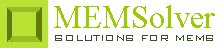PIZOACT,pcoef,area,thc,volt,ymod,sel
Longitudinal piezoelectric actuator

pcoef     longitudinal piezoelectric coefficient in C/m2
area      area of the electrode or piezo plate in µm2
thc         thickness of the piezoelectric element in µm
volt       applied voltage in Volt
ymod    Young's modulus of the material in GPa
sel         number denoting the selected result.
Use 1 for actuator displacement, 2 for actuation force and 3 for electric field strength

Notes

Some materials generate an electric field when subjected to mechanical deformation or changes dimension when voltage is applied. This effect is called piezoelectric effect. Piezo actuators utilize the deformation of the piezoelectric material when an electric field is applied. The minute control in the actuator displacement and large mechanical load capacity possible in the case of piezoelectric actuators make them suitable in a variety of applications. Piezoelectric actuation does not consume dc power and also can provide both positive and negative force unlike capacitive actuation. On the flip side, piezoelectrically induced strains and displacement are small and requires large voltage to obtain a sufficient stroke.

When the applied electric field is parallel to the actuator displacement, the actuator is described to be in longitudinal or linear configuration. The longitudinal piezoelectric coefficient couples the parallel stress and electric field. Similar actuators are used in piezoelectric flow control valves, inkjet printer heads, piezoelectric relays and switches etc.

Stacking actuators by gluing several individual actuators together will generate N times the displacement than that is possible with a single actuator where N is the number of actuators in the stack. This allows to harness the full potential of piezoelectric effect at low voltages.

The actuator displacement and the generated force are proportional to the applied voltage for any given configuration. The values calculated are for a single stack actuator. The actuator displacement is independent of the piezoelement dimensions.

The plot shows the actuator force for a single stack actuator for applied voltage ranging from -500V to +500V.

Assumptions

-The strain in the transducer is assumed to be uniform.
-The strains and electric fields in non-longitudinal directions are assumed to be zero.
-The piezoelectric element is free to deform.